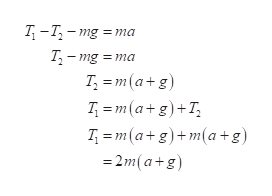Question
1 views

Two blocks each of mass m are fastened
to the top of an elevator as in Figure
P4.56. The elevator has an upward
acceleration a. The strings have negligible
mass. (a) Find the tensions T1
and T2 in the upper and lower strings in
terms of m, a, and g. (b) Compare the two tensions and determine which string would break first if
a is made sufficiently large. (c) What are the tensions if the
cable supporting the elevator breaks?

check_circle

Step 1

a)

The free body diagram is given,

From the diagram,help_outlineImage TranscriptioncloseT -T, - mg = ma T- mg = T; = m(a+g) T = m(a+g)+T, T = m(a+g)+m(a+g) = 2m(a+g) fullscreen
Step 2

The tension on the upper string

Step 3

The tension on the ...

### Want to see the full answer?

See Solution

#### Want to see this answer and more?

Solutions are written by subject experts who are available 24/7. Questions are typically answered within 1 hour.*

See Solution
*Response times may vary by subject and question.
Tagged in

### Physics